# Data Literacy Cube: Graph Data with Antarctic's Contribution to Sea Level Rise Graph

### Overview

Use the Data Literacy Cubes to guide students’ exploration of data to enrich their observations and inferences.  This is a flexible resource that may be used with a variety of graphical representations of data.  This activity requires a graph for students to evaluate.

For the purposes of this lesson, students will analyze Antarctic Ice Sheet Contribution to Global Sea Level.

### Materials Required

Teacher Preparation:

Print copies of the cube on cardstock and cut out.  Assemble the cube with glue. Note: consider laminating after you cut these out for multiple uses. (Gaming dice may be substituted for the cubes.) Also, print off copies of the differentiated Graph Cube Questions. Distribute to students for group or independent work.

### Procedure

1. Distribute one Graph Cube per group (or die), as well as the related Graph Cube Questions sheets and the graph.
2. Students roll the cube and find the matching question on the Graph Cube Question sheet.
3. Answer one question found under matching question on a sheet of paper, labeling the question with the number and letter of the question.
4. Repeat Steps 2-4 until at least 10 are answered.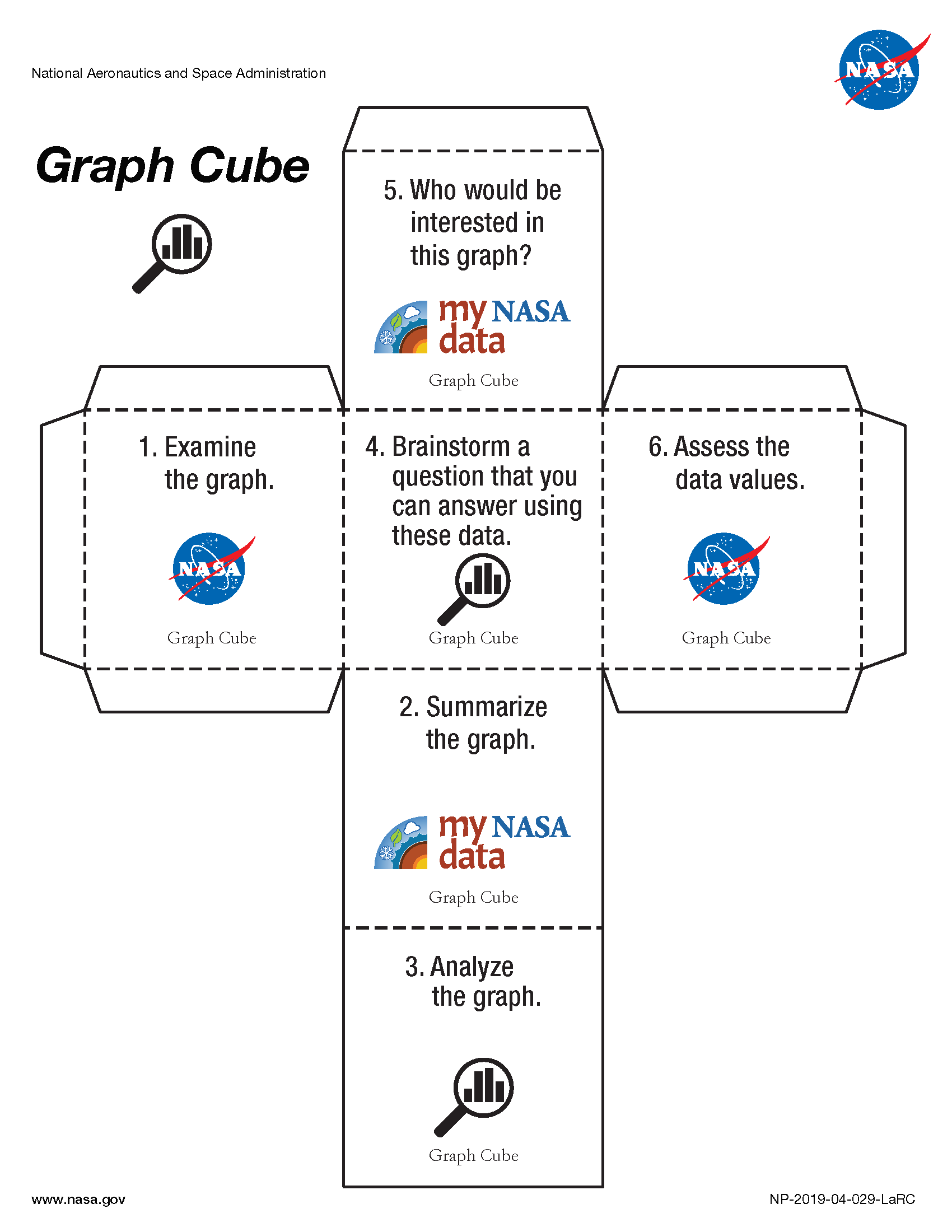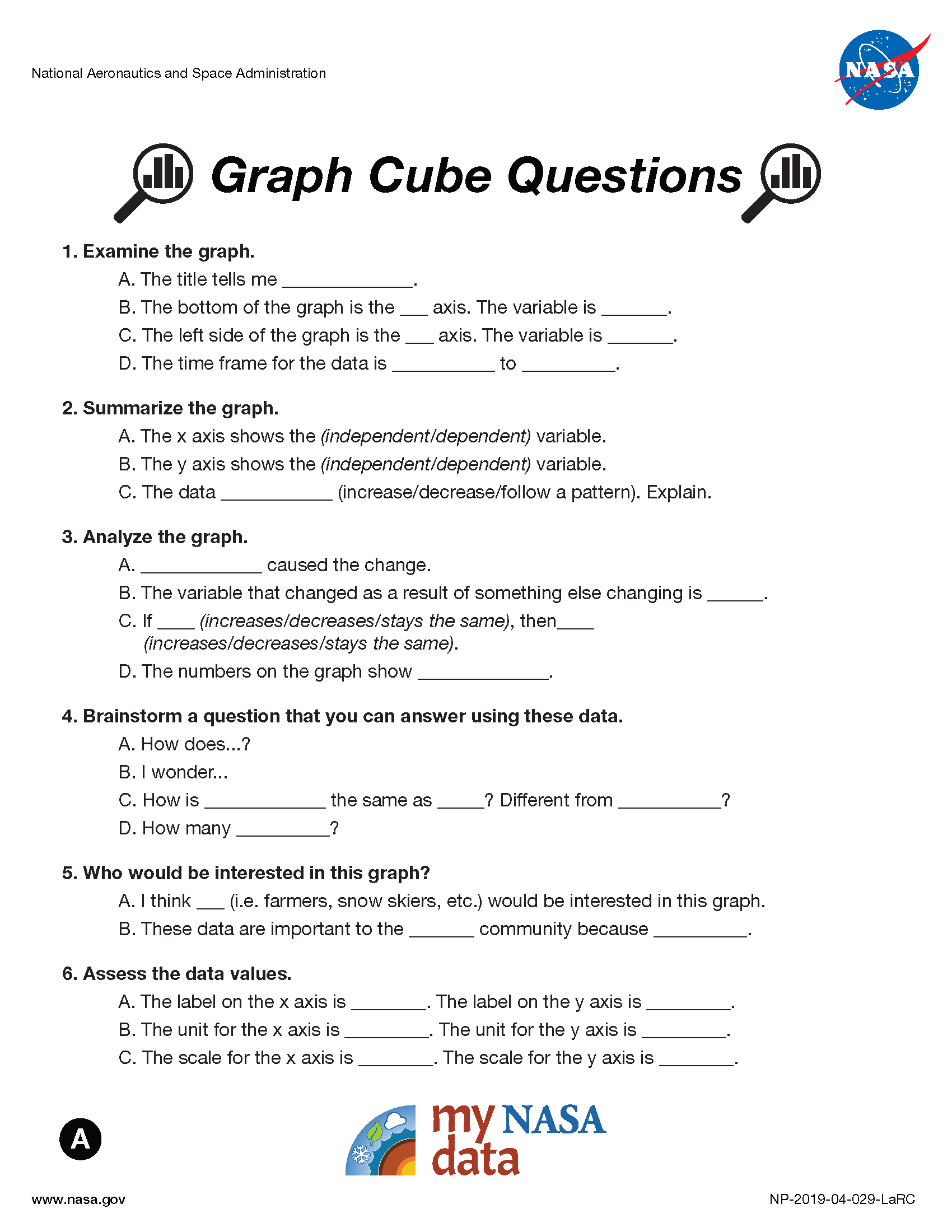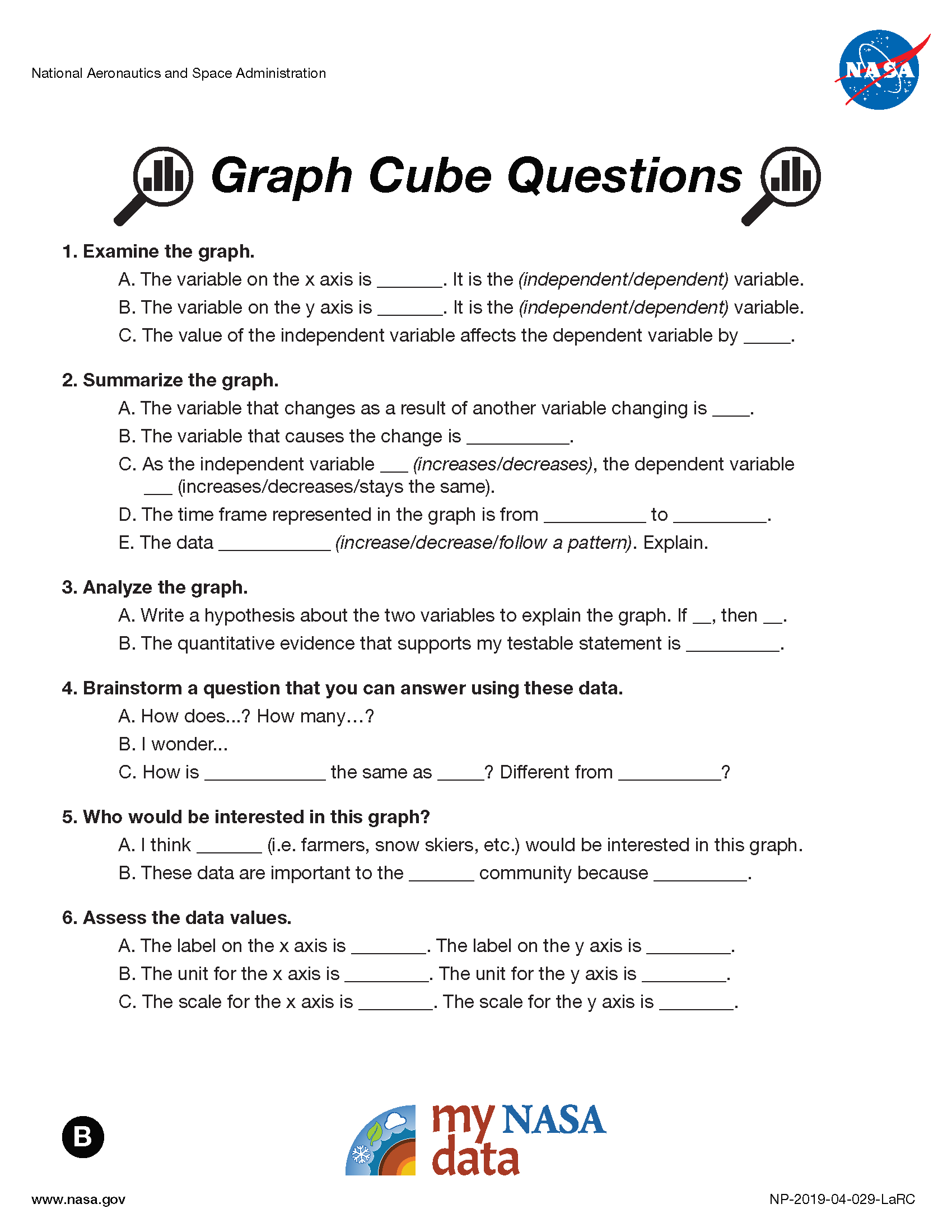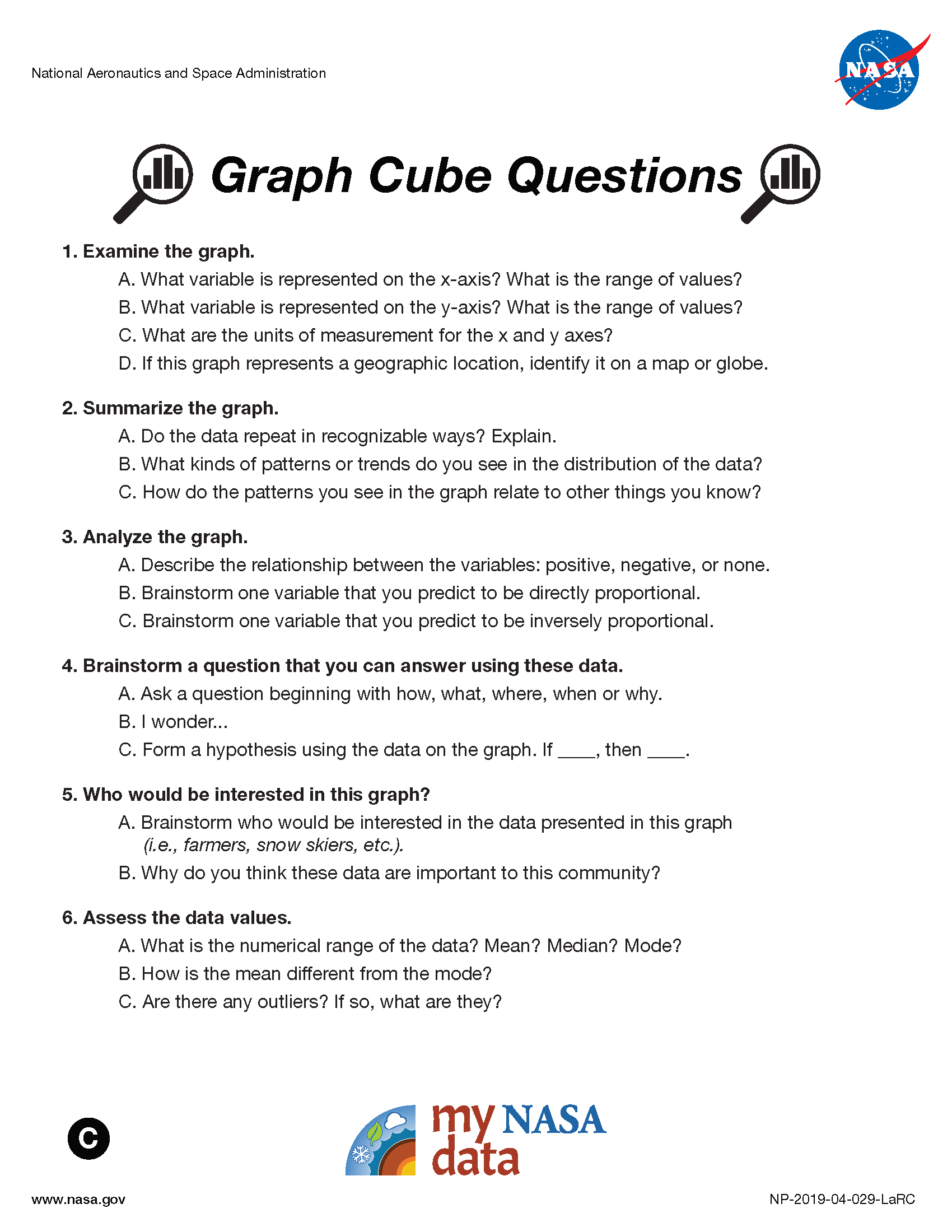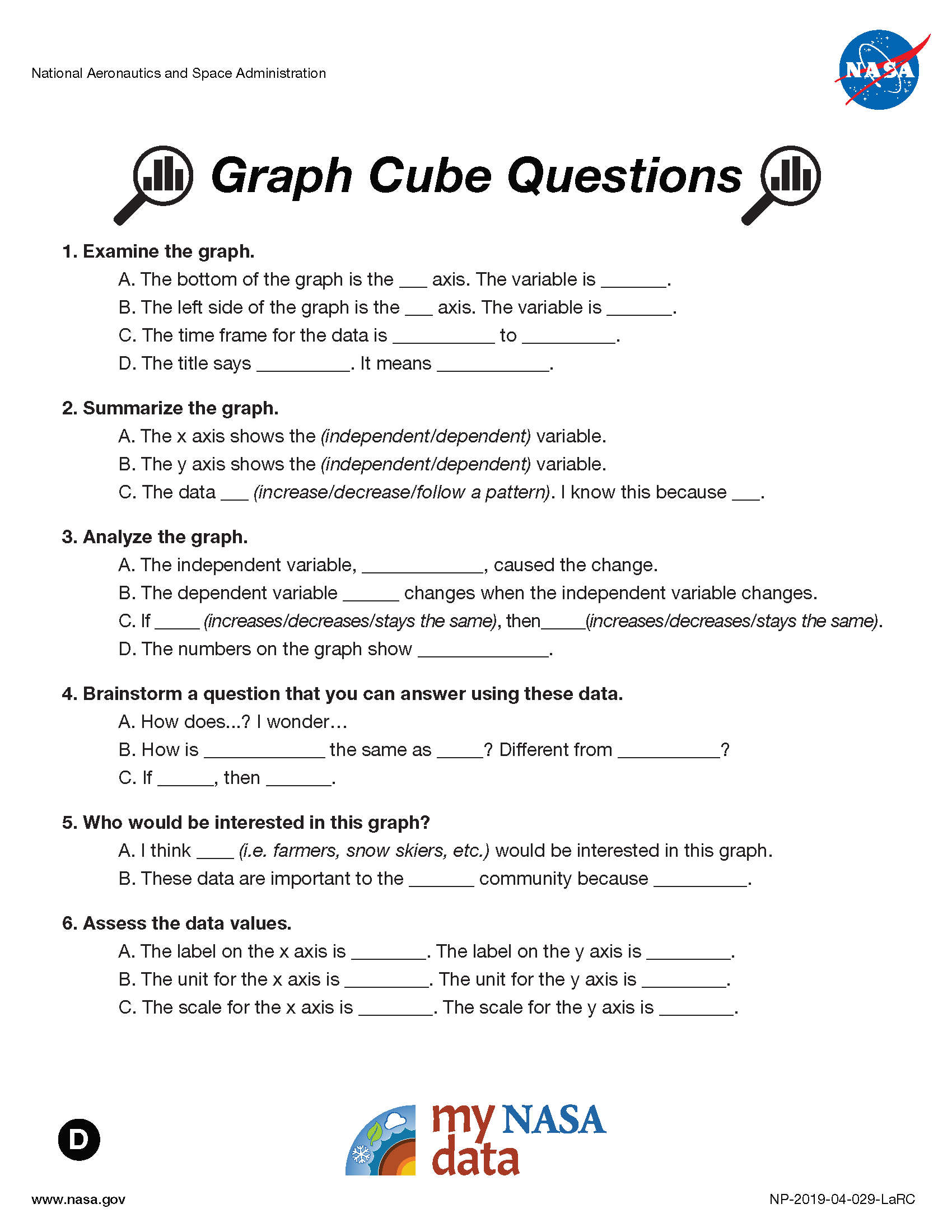• observe and interpret physical characteristics of the Earth System using graphs of NASA data
• write a claim about the variables in the graph
• analyze how the phenomena changes of time and space
• characterize the independent and dependent variables
• brainstorm the phenomena connects to other parts of the Earth System
• How are the data represented in the graph?
• How do we identify a change in these data?
• How does a change in the independent variable affect the other variable?
• What relationship do you claim exists among these variables?

• Standalone Lesson (no technology required)

### Student Resources

Student Resources